# How to use for loop in awk command

Loop is used to execute some statements multiple times. The iteration of the loop based on the termination condition or the number of the array elements. Three types of loops are supported by any programming language. For loop is one of them. For loop can be declared by two ways. Simple for loop contains three parts.  And another for loop is for-in loop that is used to iterate any list of data or array. This tutorial shows the use of these two types of loops in awk command by using various examples.

## Syntax:

1. for loop declaration:
for (initialization; termination condition; increment/decrement) {
statements
}

First part is used to initialize the variable for starting for loop. The second part contains the termination condition to control the iteration of the loop and the loop will continue until the condition is true. The last part will increment or decrement the initialization variable based on the first part.

1. for-in declaration
for (variable in array/list) {
statements
}

for-in loop is used to do those tasks where the number of iteration of the loop is not fixed. for-in loop is mainly used to parse an array or list of data. The loop reads each data from the array or list and stores the value to a variable in each iteration.

### Example-1: Using simple for loop

A simple for loop is used in the following script. Here, counter variable is initialized by 10 and the loop will terminate when the value of counter is less than 5. The script will print the counter values from 10 to 5. Run the command from the terminal.

\$ echo | awk '{ for (counter = 10; counter >= 5; counter--)
print "Running for ",counter, " times.","\n"; }'

Output:

The following output will appear after executing the command.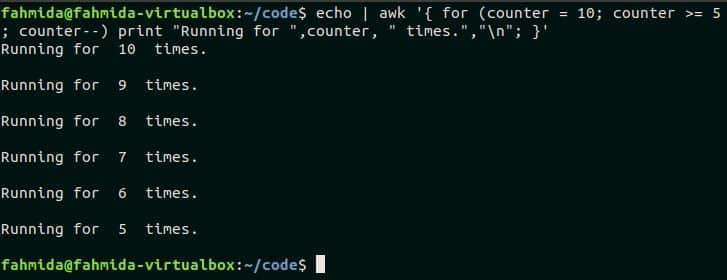### Example-2: Using for-in loop to read an array

An array named Customer is declared in the following script where the customer’s id is set as an array index and the customer’s name is set as array value. for-in loop is used here to iterate each index from the array and print the customer’s name. Run the script from the terminal.

\$ echo | awk 'BEGIN {Customer["4587"] = "Neil Johnson";
Customer["8953"] = "Ella binte Nazir";
Customer["3455"] = "Bruce Hyslop";
Customer["6335"] = "Isabella";

}END { for (i in Customer)
print  "The name of ", i, " is " ,Customer[i]
}'

Output:

The following output will appear after executing the script.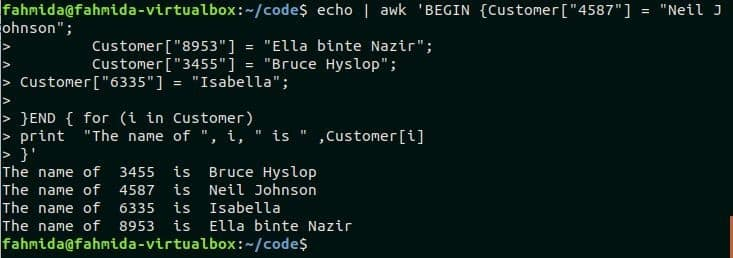### Example-3: Using nested for loop

When a for loop is declared under another for loop then it is called nested for loop. The outer loop that is used in this script will iterate for 2 times and the inner loop will iterate for 3 times. So, the print statement will execute for, (2X3=6) 6 times. Run the script from the terminal.

\$ echo | awk 'END{ for (i=1; i<=2; i++)
for (j=1; j<=3; j++)
print  "Iterating row ", i," and column ", j,"\n";}'

Output:

The following output will appear after running the script.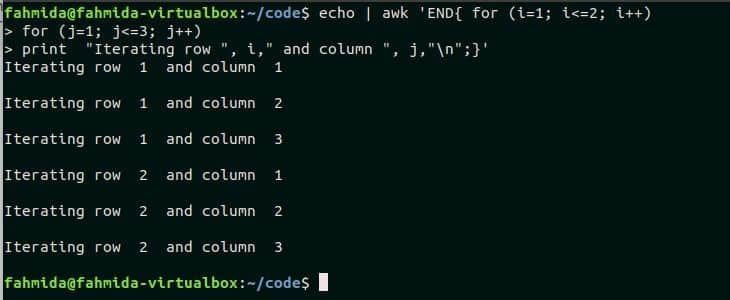### Example-4: Using for loop with break statement

break statement is used in any loop to terminate the loop before reaching the termination condition based on the particular condition. In the following script, for loop will start from 3 and it will terminate when the value of i is greater than 20. But when the value of i will be equal to the variable num then the if condition will true and the loop will terminate for the break statement.

\$ echo | awk 'BEGIN{ num=7; }END{ for (i=3; i<=20; i++) {
if( i == num) break;
else
print  "Current value of i =",i ,"\n";}}'

Output:

Here, if condition is false for four iterations when the value of i is 3,4,5 and 6. So, the following output is printed for the four iterations.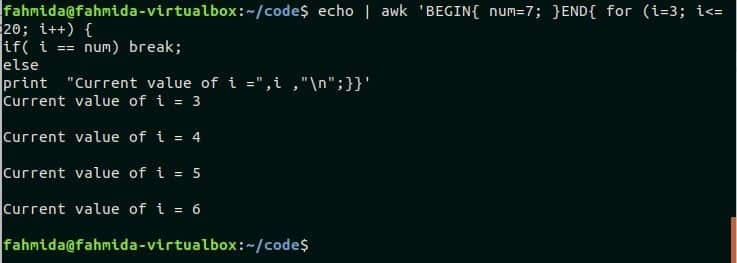### Example-5: Using for loop with continue statement

Continue statement is used in any loop to omit any statement based on any particular condition. An array named product is declared in the following script. for-in loop is used to iterate the array and check each value with “Office Software“. If the value matches then an unavailable message will print by omitting available message for continue statement, otherwise unavailable message will print. Run the script from the terminal.

\$ echo | awk 'BEGIN{product["1001"]="Antivirus";
product["1002"]="Office Software";
product["1003"]="Drawing Software";
product["1004"]="HDD";
product["1005"]="DVD";} END{ for (i in product) {
if(product[i] == "Office Software") {
print product[i]," is not available\n";
continue;
}
print product[i], " is available"  ,"\n";}}'

Output:

The following output will appear after running the script.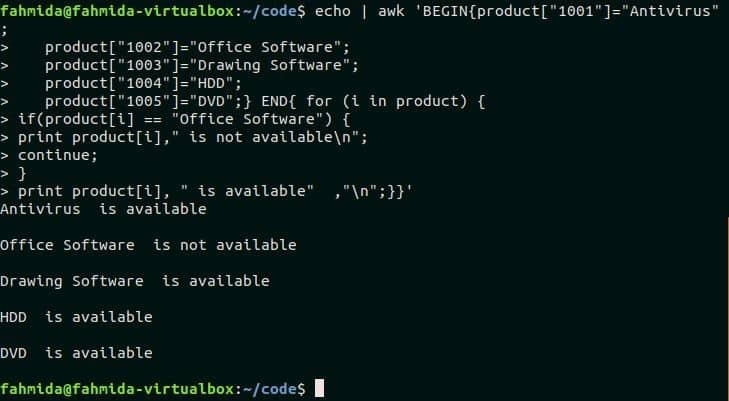### Example-6:  Using for loop in awk file

Create a text file named sales.txt and add the following content to practice this example.

sales.txt

2015 70000
2016 80000
2017 83000
2018 86000
2019 90000

Create an awk file named cal_sal.awk with the following script to calculate the total sales amount of the file sales.txt. The second field contains the yearly sales amount in the file sales.txt. In the script, the sales array will store all values of the second field and sum variable is initialized with 0 to add all values of sales array. Next, for-in loop is used to iterate each element of the sales array and add the value with the sum variable. Lastly, print statement is used to print the value of sum variable to display the total sales amount.

cal_sal.awk

{
sales[i++]=\$2;
sum=0;
}
END{
for (i in sales)
{
sum=sum+sales[i];
}
print "Total sales amount=" sum;
}

Run the following command to execute the script of cal_sal.awk file.

\$ awk -f cal_sal.awk sales.txt

Output:

There are 5 records in the sales.txt file and the sum of the sales amount is 409000 that is printed after executing the script.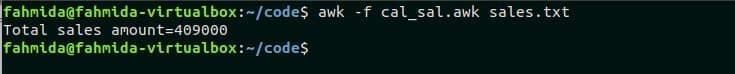#### Conclusion:

Different uses of for loop in awk command is tried to explain in this tutorial. Hope, the reader will get a clear idea on using for loop in awk script and able to use for loop properly in awk programming.